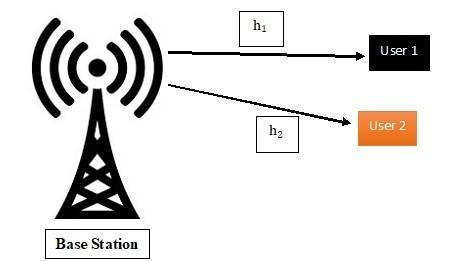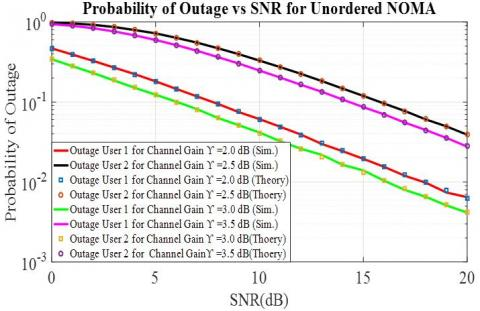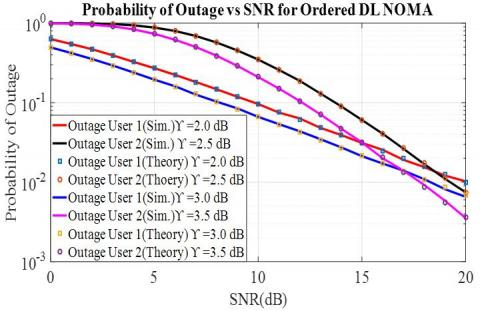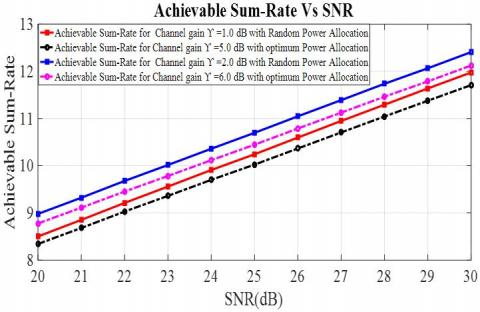# Performance Analysis of NOMA Downlink for Next- Generation 5G Network with Statistical Channel State Information

Performance Analysis of NOMA Downlink for Next- Generation 5G Network with Statistical Channel State Information
Electronics and Communication Engineering Department, National Institute of Technology Patna, Patna 800005, India

Corresponding Author Email:
indrajeet.ec16@nitp.ac.in
Page:
417-423
|
DOI:
https://doi.org/10.18280/isi.260410
10 February 2021
|
Accepted:
9 August 2021
|
Published:
31 August 2021
| Citation

OPEN ACCESS

Abstract:

Non-orthogonal multiple access (NOMA) is a compelling strategy that helps wireless networks of the fifth-generation (5G) to fulfill the diverse demands of increased fairness, high reliability, extensive connectivity, low delay and superior performance. Traditionally, NOMA network presumes perfect transmitter-side channel state information (CSI), which is almost impossible for a number of communication scenarios. Furthermore, this paper contemplates the realistic NOMA downlink method in the Rayleigh fading networks, where the statistical CSI linked with each user is known to the transmitter. We evaluate the outage probability of the proposed model and obtain a closed-form framework of the probability of outage for each user. Besides, the source can optimize the network’s achievable sum-rate for different users based on statistical CSI. The precision of our outage probability analysis and the optimum power allocation algorithm proposed are both verified by the analytical and simulation results.

Keywords:

non-orthogonal multiple access (NOMA), channel state information (CSI), outage probability, Rayleigh fading channel

1. Introduction

In the fifth-generation (5G) mobile networks, NOMA has been a promising candidate for multiuser scheduling [1, 2]. NOMA enables many users to share the same frequency-time resource by separating multiple users through distinct transmission capacity. Therefore, successive interference cancellation (SIC) on the destination side is necessary [3-5]. NOMA is capable of providing low outage probability and high sum rate as compared to traditional orthogonal multiple access (OMA), along with better user fairness . NOMA uses superposition coding to exploit the power domain and successive interference cancellation (SIC) for multiuser detection. Numerous prevailing works about NOMA have presumed perfect CSI [7-9]. However, this supposition is almost unworkable in certain communication situations, such as high-speed railway  and underwater acoustic  communications, due to significant feedback delay and quickly changing channel. Newly, NOMA network with statistical CSI have been considered in few literature [12, 13]. A power-efficient resource sharing scheme with statistical CSI for multicarrier downlink NOMA network is suggested. In the meantime, NOMA for underwater acoustic multiplexing orthogonal frequency-division (OFDM) network was examined in the Ref. . In precise, Shankar et al.  suggested that NOMA significantly outperforms traditional frequency division multiple access (FDMA) systems in terms of ergodic data rate and outage probability taking into account the fading channel of Nakagami-m. But Shankar et al.  emphasizes only on the situation of two users and lacks a closed-form study of the likelihood of each and every user outage. Many beneficial features, for example range extension, capability enhancement, and diversity benefit in wireless network, cooperative network has gained considerable attention . This enhancement in efficiency of the cooperative network is due to virtual multiple-input multiple-output (MIMO) network generated between the source and the destination employing spatially separated relay nodes . Multiuser scheduling allows the scheduler to provide limited resources to these users while properly picking a group of users who match a set of criteria . Proportional fairness (PF) scheduling is a good trade-off between system throughput and fairness for NOMA scheduling and power allocation. PF also has a high level of complexity and is unable to limit the amount of resources available since it allocates the majority of resources to users with poor channel conditions [19, 20].

In AF-based cooperation , the relay magnifies the signal upon receipt of the signal communicated by the source and then transmits it to the receiver. On the other side, the relay decodes the signal in a DF network and retransmits to the receiver the message thus received [22-24]. In this paper, we use statistical CSI to evaluate the downlink NOMA system's outage performance. The device's ergodic data rate region is calculated using the Monte Carlo (MC) technique considering the Rayleigh fading channel model. Furthermore, the closed form expression for each user's outage probability is calculated.

The remainder of this article is structured as follows. A system model is defined in section 2. Section 3 analyses the Outage Probability of the system. Optimum power allocation is discussed in Section 4. The simulation outcomes are given in Section 5 to validate the outage study. Finally, the conclusion of paper is discussed in Section 6.

2. System Model

Consider implementing the hybrid multiple access schemes  as a single-cell downlink multi-user single-input multiple-output (SIMO) system. Users are split into multiple groups in this scheme and each group comprises users L, indicated by $UE-L\,(1\le l\le L)$. In each group, the NOMA downlink is executed, while OMA is used to prevent inter-group interference. The SIC complexity can be minimized by this scheme thus improving the efficiency of the system . In addition, each user is having fixed data rate requirement in our considered scenario, dictated by his targeted service quality (QoS). We concentrate on one category in this paper adopting NOMA within two users. Figure 1 indicate base station (BS) and two users for downlink NOMA system.

## 1.jpgFigure 1. BS and two users for downlink NOMA system

## 2.jpgFigure 2. Different power levels of two users in same frequency range

The BS transmits the superimposed signal having the data of all the two users ${{u}_{1}}$ and ${{u}_{2}}$in the case of NOMA, and the signal being superimposed is given as ${{z}_{R}}$:

${{z}_{R}}=\sqrt{{{a}_{1}}{{\varphi }_{s}}}{{z}_{1}}+\sqrt{{{a}_{2}}{{p}_{s}}}{{z}_{2}}$               (1)

The superimposed signal that user $l$ receives is given as:

${{y}_{l}}={{h}_{l}}{{z}_{R}}+{{w}_{i}}$                 (2)

The superimposed signal obtained by the user $l$ can be expressed as Eq. (3), after substituting the Eq. (1) in Eq. (2):

${{y}_{l}}={{h}_{l}}\left( \sqrt{{{a}_{1}}{{\varphi }_{s}}}{{z}_{1}}+\sqrt{{{a}_{2}}{{\varphi }_{s}}}{{z}_{2}} \right)+{{w}{l}}$                (3)

where, ${{h}_{l}}\sim \mathbb{C}N(0,\,{{\rho }^{2}})$ represents the faded channel coefficient of Rayleigh. ${{a}_{1}}$and ${{a}_{2}}$ are fraction of power given to users ${{u}_{1}}$ and ${{u}_{2}}$respectively. For power constraint ${{a}_{1}}+{{a}_{2}}=1$. Here, ${{\varphi }_{s}}$is total transmit SNR.${{\varphi }_{s}}=\frac{{{P}_{T}}}{{{\rho }^{2}}}$ where ${{P}_{T}}$ is total transmit power. Figure 2 indicates the division of total power between two users in the same frequency band. ${{z}_{1}}$and ${{z}_{2}}$ are symbols of the two users. Additive white Gaussian noise with mean 0 and variance 1 is represented by${{w}_{l}}\sim N(0,\,1)$.

The signal received at User 1 is:

${{y}_{1}}={{h}_{1}}\left( \sqrt{{{a}_{1}}{{\varphi }_{s}}}{{z}_{1}}+\sqrt{{{a}_{2}}{{\varphi }_{s}}}{{z}_{2}} \right)+{{w}_{1}}$            (4)

The signal to interference noise ratio (SINR), for decoding ${{z}_{1}}$ at user 1 can be expressed as:

$\xi _{{{u}_{1}}}^{{{z}_{1}}}=\frac{{{a}_{1}}{{\varphi }_{s}}{{\left| {{h}_{1}} \right|}^{2}}}{{{a}_{2}}{{\varphi }_{s}}{{\left| {{h}_{1}} \right|}^{2}}+1}=\frac{{{a}_{1}}{{\varphi }_{s}}{{\gamma}_{1}}}{{{a}_{2}}{{\varphi }_{s}}{{\gamma }_{1}}+1},{{\gamma }_{1}}={{\left| {{h}_{1}} \right|}^{2}}$              (5)

The achievable rate for the first user is $\mathbb{S}_{{{u}_{1}}}^{{{z}_{1}}}$,

$\mathbb{S}_{{{u}_{1}}}^{{{z}_{1}}}={{\log }_{2}}\left( 1+\xi _{{{u}_{1}}}^{{{z}_{1}}} \right)={{\log }_{2}}\left( 1+\frac{{{a}_{1}}{{\varphi }_{s}}{{\gamma }_{1}}}{{{a}_{2}}{{\varphi }_{s}}{{\gamma }_{1}}+1} \right)$                   (6)

The received signal ${{y}_{2}}$ at the second user is,

${{y}_{2}}={{h}_{2}}\left( \sqrt{{{a}_{1}}{{\varphi }_{s}}}{{z}_{1}}+\sqrt{{{a}_{2}}{{\varphi }_{s}}}{{z}_{2}} \right)+{{w}_{2}}$             (7)

The SINR at the second user, for decoding ${{z}_{1}}$ is given as

$\xi _{{{u}_{2}}}^{{{z}_{1}}}=\frac{{{a}_{1}}{{\varphi }_{s}}{{\left| {{h}_{2}} \right|}^{2}}}{{{a}_{2}}{{\varphi }_{s}}{{\left| {{h}_{2}} \right|}^{2}}+1}=\frac{{{a}_{1}}{{\varphi }_{s}}{{\gamma }_{2}}}{{{a}_{2}}{{\varphi }_{s}}{{\gamma }_{2}}+1}$       (8)

The Eq. (7) can be reduced, after decoding the signal of first user and successfully eliminating its interference.

${{y}_{2}}={{h}_{2}}\sqrt{{{a}_{2}}{{\varphi }_{s}}}{{z}_{2}}+{{w}_{2}}$            (9)

At second user SINR, for decoding ${{z}_{2}}$signal is expressed as:

$\xi _{{{u}_{2}}}^{{{z}_{2}}}={{a}_{2}}{{\varphi }_{s}}{{\left| {{h}_{2}} \right|}^{2}}={{a}_{2}}{{\varphi }_{s}}{{\gamma }_{2}}$        (10)

The total sum-rate for the NOMA downlink is expressed as:

${{\mathbb{S}}_{D}}=\mathbb{S}_{{{u}_{1}}}^{{{z}_{1}}}+\mathbb{S}_{{{u}_{2}}}^{{{z}_{2}}}$$={{\log }_{2}}\left( 1+\xi _{{{u}_{1}}}^{{{z}_{1}}} \right)+{{\log }_{2}}\left( 1+\xi _{{{u}_{2}}}^{{{z}_{2}}} \right)={{\log }_{2}}\left( 1+\frac{{{a}_{1}}{{\varphi }_{s}}{{\gamma }_{1}}}{{{a}_{2}}{{\varphi }_{s}}{{\gamma }_{1}}+1} \right)+{{\log }_{2}}\left( 1+{{a}_{2}}{{\varphi }_{s}}{{\gamma }_{2}} \right) (11) 3. Outage Probability Analysis The probability that a certain data rate will not be supported owing to fluctuating channel conditions is known as the outage probability of a transmission channel in Information Theory. The outage probability is based on the possibility that the data rate will be lower than the required threshold data rate. Let the required threshold be {{\hat{\Re }}_{1}}. For outage to occur, the required data rate must be less than the threshold {{\hat{\Re }}_{1}}. From Eq. (11), we can easily see that, \mathbb{S}_{{{u}_{1}}}^{{{z}_{1}}}<{{\hat{\Re }}_{1}}. In this paper we will calculate the outage probability for unordered (fixed) and ordered NOMA Downlink. 3.1 Outage probability analysis for unordered Downlink NOMA An outage is a condition in which the user data rate is smaller than the required data rate . Let us suppose {{\hat{\Re }}_{1}} and {{\hat{\Re }}_{2}} be the required data rate for first user UE-1 and second user UE-2. 3.1.1 For first user UE-1 The sum-rate must be less than the required data rate {{\hat{\Re }}_{1}}, for outage to occurs. Using Eq. (11), we have, \mathbb{S}_{{{u}_{1}}}^{{{z}_{1}}}={{\log }_{2}}\left( 1+\xi _{{{u}_{1}}}^{{{z}_{1}}} \right)<{{\hat{\Re }}_{1}} (12) Putting Eq. (5) in above equation and solving we have, \xi _{{{u}_{1}}}^{{{z}_{1}}}=\frac{{{a}_{1}}{{\varphi }_{s}}{{\gamma }_{1}}}{{{a}_{2}}{{\varphi }_{s}}{{\gamma }_{1}}+1}<{{2}^{{{{\hat{\Re }}}_{1}}}}-1={{\Re }_{1}} (13) {{\gamma }_{1}}<\frac{{{\Re }_{1}}}{\left( {{a}_{1}}-{{a}_{2}}{{\Re }_{1}} \right){{\varphi }_{s}}} (14) Here, {{\gamma }_{1}} is demarcated as {{\gamma }_{1}}={{\left| {{h}_{1}} \right|}^{2}} and is distributed as: {{f}_{\gamma }}\left( {{\gamma }_{1}} \right)=\frac{1}{\chi _{1}^{2}}{{e}^{\frac{-{{\gamma }_{1}}}{\chi _{1}^{2}}}},{{\gamma }_{1}}\ge 0and E\left\{ {{\gamma }_{1}} \right\}=\chi _{1}^{2} The probability of outage first userUE-1. P\left\{ {{\gamma }_{1}}<\frac{{{\Re }_{1}}}{\left( {{a}_{1}}-{{a}_{2}}{{\Re }_{1}} \right){{\varphi }_{s}}} \right\}$$=\int\limits_{0}^{\frac{{{\Re }_{1}}}{\left( {{a}_{1}}-{{a}_{2}}{{\Re }_{1}} \right){{\varphi }_{s}}}}{{{f}_{\gamma }}\left( {{\gamma }_{1}} \right)d{{\gamma }_{1}}}=1-\exp \left( -\frac{{{\Re }_{1}}}{\chi _{1}^{2}{{\varphi }_{s}}\left( {{a}_{1}}-{{a}_{2}}{{\Re }_{1}} \right)} \right)$                  (15)

The probability of outage at second user$UE-2$.

At second user$UE-2$ decoding of message fails if either decoding of ${{z}_{1}}$ or ${{z}_{2}}$ fails.

3.1.2 For second user $UE-2$

Outage probability at second user$UE-2$ is:

$P\left\{ \mathbb{S}_{{{u}_{2}}}^{{{z}_{1}}}<{{{\hat{\Re }}}_{1}}\bigcup \,\,\mathbb{S}_{{{u}_{2}}}^{{{z}_{2}}}<{{{\hat{\Re }}}_{2}} \right\}$                (16)

$\mathbb{S}_{{{u}_{2}}}^{{{z}_{1}}}<{{\hat{\Re }}_{1}}\,\,\Rightarrow {{\log }_{2}}\left( 1+\xi _{{{u}_{2}}}^{{{z}_{1}}} \right)<{{\hat{\Re }}_{1}}$          (17)

After solving the above equation and using Eq. (8), we have:

$\Rightarrow \xi _{{{u}_{2}}}^{{{z}_{1}}}=\frac{{{a}_{1}}{{\varphi }_{s}}{{\gamma }_{2}}}{{{a}_{2}}{{\varphi }_{s}}{{\gamma }_{2}}+1}<{{2}^{{{{\hat{\Re }}}_{1}}}}-1={{\Re }_{1}}$$\Rightarrow {{\gamma }_{2}}<\frac{{{\Re }_{1}}}{\left( {{a}_{1}}-{{a}_{2}}{{\Re }_{1}} \right){{\varphi }_{s}}} (18) Also, \mathbb{S}_{{{u}_{2}}}^{{{z}_{2}}}<{{\hat{\Re }}_{2}}\,\,\Rightarrow {{\log }_{2}}\left( 1+\xi _{{{u}_{2}}}^{{{z}_{2}}} \right)<{{\hat{\Re }}_{2}} (19) Solving the above equation and using Eq. (10), we have: \Rightarrow \xi _{{{u}_{2}}}^{{{z}_{2}}}={{a}_{2}}{{\varphi }_{s}}{{\gamma }_{2}}<{{2}^{{{{\hat{\Re }}}_{2}}}}-1={{\Re }_{2}}$$\Rightarrow {{\gamma }_{2}}<\frac{{{\Re }_{2}}}{{{a}_{2}}{{\varphi }_{s}}}$                 (20)

Outage probability at second user$UE-2$is given as$P\left\{ \mathbb{S}_{{{u}_{2}}}^{{{z}_{1}}}<{{{\hat{\Re }}}_{1}}\bigcup \,\,\mathbb{S}_{{{u}_{2}}}^{{{z}_{2}}}<{{{\hat{\Re }}}_{2}} \right\}$, relating the two inequalities outage occurs if

${{\gamma }_{2}}<\max \left\{ \frac{{{\Re }_{1}}}{\left( {{a}_{1}}-{{a}_{2}}{{\Re }_{1}} \right){{\varphi }_{s}}},\frac{{{\Re }_{2}}}{{{a}_{2}}{{\varphi }_{s}}} \right\}$                       (21)

PDF of above Eq. (21),${{\gamma }_{2}}$is given as ${{f}_{\gamma }}\left( {{\gamma }_{2}} \right)=\frac{1}{\chi _{2}^{2}}{{e}^{\frac{-{{\gamma }_{2}}}{\chi _{2}^{2}}}},{{\gamma }_{2}}\ge 0$. The outage probability for decoding message at second user$UE-2$is given as:

$P\left\{ {{\gamma }_{2}}<\max \left\{ \frac{{{\Re }_{1}}}{\left( {{a}_{1}}-{{a}_{2}}{{\Re }_{1}} \right){{\varphi }_{s}}},\frac{{{\Re }_{2}}}{{{a}_{2}}{{\varphi }_{s}}} \right\} \right\}$$=\int\limits_{0}^{\max \left\{ \frac{{{\Re }_{1}}}{\left( {{a}_{1}}-{{a}_{2}}{{\Re }_{1}} \right){{\varphi }_{s}}},\frac{{{\Re }_{2}}}{{{a}_{2}}{{\varphi }_{s}}} \right\}}{{{f}_{\gamma }}\left( {{\gamma }_{2}} \right)d{{\gamma }_{2}}}$$=1-\exp \left( -\frac{1}{\chi _{2}^{2}}\max \left\{ \frac{{{\Re }_{1}}}{\left( {{a}_{1}}-{{a}_{2}}{{R}_{1}} \right){{\varphi }_{s}}},\frac{{{\Re }_{2}}}{{{a}_{2}}{{\varphi }_{s}}} \right\} \right)$                  (22)

3.2 Outage probability analysis for ordered NOMA Downlink

In ordered NOMA, the weaker user is decoded first, who has channel gain

${{\hat{\gamma }}_{1}}={{\left| {{{\hat{h}}}_{1}} \right|}^{2}}=\min \left\{ {{\left| {{h}_{1}} \right|}^{2}},{{\left| {{h}_{2}} \right|}^{2}} \right\}=\min \left\{ {{\gamma }_{1}},{{\gamma }_{2}} \right\}$                (23)

The stronger user is decoded next, with channel gain

${{\hat{\gamma }}_{2}}={{\left| {{{\hat{h}}}_{2}} \right|}^{2}}=\max \left\{ {{\left| {{h}_{1}} \right|}^{2}},{{\left| {{h}_{2}} \right|}^{2}} \right\}=\max \left\{ {{\gamma }_{1}},{{\gamma }_{2}} \right\}$               (24)

The channel gain for first user$UE-1$

${{\hat{\gamma }}_{1}}={{\left| {{{\hat{h}}}_{1}} \right|}^{2}}=\min \left\{ {{\left| {{h}_{1}} \right|}^{2}},{{\left| {{h}_{2}} \right|}^{2}} \right\}$         (25)

The Received signal for the ordered NOMA,

${{y}_{l}}={{\hat{h}}_{l}}\left( \sqrt{{{a}_{1}}{{\varphi }_{s}}}{{{\hat{z}}}_{1}}+\sqrt{{{a}_{2}}{{\varphi }_{s}}}{{{\hat{z}}}_{2}} \right) +{{w}_{l}}$              (26)

3.2.1 For first user $UE-1$

The outage probability for ordered first user $UE-1$is:

${{P}_{out}}=P\left( \mathbb{S}_{{{{\hat{u}}}_{1}}}^{{{z}_{1}}}<{{{\hat{\Re }}}_{1}} \right)=P\left( \xi _{{{u}_{1}}}^{{{z}_{1}}}=\frac{{{a}_{1}}{{\varphi }_{s}}{{{\hat{\gamma }}}_{1}}}{{{a}_{2}}{{\varphi }_{s}}{{{\hat{\gamma }}}_{1}}+1}<{{2}^{{{{\hat{\Re }}}_{1}}}}-1={{\Re }_{1}} \right)=P\left( {{{\hat{\gamma }}}_{1}}<\frac{{{\Re }_{1}}}{{{\varphi }_{s}}({{a}_{1}}-{{a}_{2}}{{\Re }_{1}})} \right)$             (27)

The probability density function of ${{\gamma }_{1}}\,and\,{{\gamma }_{2}}$ is given as${{f}_{{{\gamma }_{1}}}}\left( {{\gamma }_{1}} \right)=\frac{1}{\chi _{1}^{2}}{{e}^{-\frac{{{\gamma }_{1}}}{\chi _{1}^{2}}}}$ and ${{f}_{{{\gamma }_{2}}}}\left( {{\gamma }_{2}} \right)=\frac{1}{\chi _{2}^{2}}{{e}^{-\frac{{{\gamma }_{2}}}{\chi _{2}^{2}}}}$ respectively.

The cumulative distribution function for first user$UE-1$,

${{F}_{{{{\hat{C}}}_{1}}}}\left( {{{\hat{\gamma }}}_{1}} \right)=P\left( {{{\hat{C}}}_{1}}\le {{{\hat{\gamma }}}_{1}} \right)=1-P\left( {{{\hat{C}}}_{1}}>{{{\hat{\gamma }}}_{1}} \right)=1-P\left( \min \,\left\{ {{C}_{1}},{{C}_{2}} \right\} \right)$               (28)

The above Eq. (28) can be simplified as:

{{F}_{{{{\hat{C}}}_{1}}}}\left( {{{\hat{\gamma }}}_{1}} \right)=1-P\left( \min \,\left\{ {{C}_{1}},{{C}_{2}} \right\}>{{{\hat{\gamma }}}_{1}} \right)=1-P\left( {{C}_{1}}>{{{\hat{\gamma }}}_{1}},\,{{C}_{2}}>{{{\hat{\gamma }}}_{2}} \right)=1-P\left( {{C}_{1}}>{{{\hat{\gamma }}}_{1}} \right)P\left( {{C}_{2}}>{{{\hat{\gamma }}}_{2}} \right) (29) P\left( {{C}_{1}}>{{{\hat{\gamma }}}_{1}} \right)={{e}^{-\frac{{{{\hat{\gamma }}}_{1}}}{\chi _{1}^{2}}}}\,\,and\,P\left( {{C}_{2}}>{{{\hat{\gamma }}}_{1}} \right)={{e}^{-\frac{{{{\hat{\gamma }}}_{1}}}{\chi _{2}^{2}}}} (30) The above Eq. (29) can be simplified using Eq. (30), {{F}_{{{{\hat{C}}}_{1}}}}\left( {{{\hat{\gamma }}}_{1}} \right)=1-{{e}^{-\frac{{{{\hat{\gamma }}}_{1}}}{\chi _{1}^{2}}}}\times {{e}^{-\frac{{{{\hat{\gamma }}}_{1}}}{\chi _{2}^{2}}}}=1-{{e}^{-{{{\hat{\gamma }}}_{1}}\left( \frac{1}{\chi{1}^{2}}+\frac{1}{\chi _{2}^{2}} \right)}} (31) We can find easily find pdf after differentiating the above Eq. (31), {{f}_{{{{\hat{C}}}_{1}}}}\left( {{{\hat{\gamma }}}_{1}} \right)=\left( \frac{1}{\chi _{1}^{2}}+\frac{1}{\chi _{2}^{2}} \right){{e}^{-{{{\hat{\gamma }}}_{1}}\left( \frac{1}{\chi _{1}^{2}}+\frac{1}{\chi _{2}^{2}} \right)}}=\frac{1}{\chi _{3}^{2}}{{e}^{-\frac{{{{\hat{\gamma }}}_{1}}}{\chi _{3}^{2}}}} (32) Here \frac{1}{\chi _{3}^{2}}=\frac{1}{\chi _{1}^{2}}+\frac{1}{\chi _{2}^{2}}. Outage probability will be: {{P}_{out}}=\int_{0}^{\frac{{{\Re }_{1}}}{{{\varphi }_{s}}\left( {{a}_{1}}-{{a}_{2}}{{\Re }_{1}} \right)}}{\frac{1}{\chi _{3}^{2}}}{{e}^{-\frac{{{{\hat{\gamma }}}_{1}}}{\chi _{3}^{2}}}}d{{\hat{\gamma }}_{1}}=1-\exp \left( -\frac{{{\Re }_{1}}}{\chi _{3}^{2}{{\varphi }_{s}}\left( {{a}_{1}}-{{a}_{2}}{{\Re }_{1}} \right)} \right) (33) 3.2.2 For second user UE-2 The outage probability for ordered second user UE-2 is {{\hat{\gamma }}_{2}}={{\left| {{{\hat{h}}}_{2}} \right|}^{2}}=\max \left\{ {{\left| {{h}_{1}} \right|}^{2}},{{\left| {{h}_{2}} \right|}^{2}} \right\}=\max \left\{ {{\gamma }_{1}},{{\gamma }_{2}} \right\} (34) {{F}_{{{{\hat{C}}}_{2}}}}\left( {{{\hat{\gamma }}}_{2}} \right)=P\left( {{{\hat{C}}}_{2}}\le {{{\hat{\gamma }}}_{2}} \right)=P\left( \max \left\{ {{\left| {{h}_{1}} \right|}^{2}},{{\left| {{h}_{2}} \right|}^{2}} \right\}\le {{{\hat{\gamma }}}_{2}} \right)=P\left( {{C}_{1}}\le {{{\hat{\gamma }}}_{2}} \right)P\left( {{C}_{2}}\le {{{\hat{\gamma }}}_{2}} \right) =\left( 1-\exp \left( -\frac{{{{\hat{\gamma }}}_{2}}}{\chi _{1}^{2}} \right) \right)\left( 1-\exp \left( -\frac{{{{\hat{\gamma }}}_{2}}}{\chi _{2}^{2}} \right) \right)=1-{{e}^{-\frac{{{{\hat{\gamma }}}_{2}}}{\chi _{1}^{2}}}}-{{e}^{-\frac{{{{\hat{\gamma }}}_{2}}}{\chi _{2}^{2}}}}+{{e}^{-\frac{{{{\hat{\gamma }}}_{2}}}{\chi _{3}^{2}}}} (35) Here \frac{1}{\chi _{3}^{2}}=\frac{1}{\chi _{1}^{2}}+\frac{1}{\chi _{2}^{2}}. The outage probability for ordered second user UE-2is: {{P}_{out}}=P\left( \frac{{{a}_{1}}{{\varphi }_{s}}{{\left| {{{\hat{h}}}_{2}} \right|}^{2}}}{1+{{a}_{2}}{{\varphi }_{s}}{{\left| {{{\hat{h}}}_{2}} \right|}^{2}}}\ge {{\Re }_{1}}\,U\,{{a}_{2}}{{\varphi }_{s}}{{\left| {{{\hat{h}}}_{2}} \right|}^{2}}\ge {{\Re }_{2}} \right)=P\left( {{{\hat{\gamma }}}_{2}}<\psi \right) =P\left( {{{\hat{\gamma }}}_{2}}<\max \,\left\{ \frac{{{\Re }_{1}}}{{{\varphi }_{s}}({{a}_{1}}-{{a}_{2}}{{\Re }_{1}})},\frac{{{\Re }_{2}}}{{{a}_{2}}{{\varphi }_{s}}} \right\} \right)={{F}_{{{{\hat{C}}}_{2}}}}\left( \psi \right) (36) where, \psi =\max \,\left\{ \frac{{{\Re }_{1}}}{{{\varphi }_{s}}({{a}_{1}}-{{a}_{2}}{{\Re }_{1}})},\frac{{{\Re }_{2}}}{{{a}_{2}}{{\varphi }_{s}}} \right\}. {{P}_{out}}=1-\exp \left( -\frac{\psi }{\chi _{1}^{2}} \right)-\exp \left( -\frac{\psi }{\chi _{2}^{2}} \right)+\exp \left( -\frac{\psi }{\chi _{3}^{2}} \right) (37) 4. Optimum Power Allocation The Sum Capacity of downlink NOMA is given as: \begin{align} & \mathbb{S}_{{{u}_{1}}}^{{{z}_{1}}}+\mathbb{S}_{{{u}_{2}}}^{{{z}_{2}}}={{\log }_{2}}\left( 1+\frac{{{a}_{1}}{{\varphi }_{s}}{{\gamma }_{1}}}{{{a}_{2}}{{\varphi }_{s}}{{\gamma }_{1}}+1} \right)+{{\log }_{2}}\left( 1+{{a}_{2}}{{\varphi }_{s}}{{\gamma }_{2}} \right) \\ & \,\,\,\,\,\,\,\,\,\,\,\,\,\,\,\,\,\,\,={{\log }_{2}}\left( \frac{1+{{\varphi }_{s}}{{\gamma }_{1}}}{1+{{a}_{2}}{{\varphi }_{s}}{{\gamma }_{1}}} \right)+{{\log }_{2}}\left( 1+{{a}_{2}}{{\varphi }_{s}}{{\gamma }_{2}} \right) \\\end{align}={{\log }_{2}}\left( \left( \underbrace{{{\varphi }_{s}}{{\gamma }_{1}}+1}_{cons\tan t} \right)\left( \frac{1+{{a}_{2}}{{\varphi }_{s}}{{\gamma }_{2}}}{1+{{a}_{2}}{{\varphi }{s}}{{\gamma }_{1}}} \right) \right)                    (38)

The above sum capacity can be maximizing only if $\frac{1+{{a}_{2}}{{\varphi }_{s}}{{\gamma }_{2}}}{1+{{a}_{2}}{{\varphi }_{s}}{{\gamma }_{1}}}$will have maximum value for optimal power allocation.

$\frac{1+a_{2} \varphi_{s} \gamma_{2}}{1+a_{2} \varphi_{s} \gamma_{1}}=1+\frac{\left(\gamma_{2}-\gamma_{1}\right)}{\gamma_{1}+\frac{1}{a_{2} \varphi_{s}}}$              (39)

The maximum sum-rate occurs for maximum value of ${{a}_{2}}$, However, this has to be in the feasible region,

$\mathbb{S}_{{{u}_{1}}}^{{{z}_{1}}}\ge {{\hat{\Re }}_{1}}\,\,\Rightarrow {{\log }_{2}}\left( 1+\xi _{{{u}_{1}}}^{{{z}_{1}}} \right)\ge {{\hat{\Re }}_{1}}$$\Rightarrow \xi _{{{u}_{1}}}^{{{z}_{1}}}=\frac{{{a}_{1}}{{\varphi }_{s}}{{\gamma }_{1}}}{{{a}_{2}}{{\varphi }_{s}}{{\gamma }_{1}}+1}\ge {{2}^{{{{\hat{\Re }}}_{1}}}}-1={{\Re }_{1}}$

$\Rightarrow \left( 1-{{a}_{2}} \right){{\varphi }_{s}}{{\gamma }_{1}}\ge \left( 1+{{a}_{2}}{{\varphi }_{s}}{{\gamma }_{1}} \right){{\Re }_{1}}$

$\Rightarrow {{\varphi }_{s}}{{\gamma }_{1}}-{{\Re }_{1}}\ge {{a}_{2}}{{\varphi }_{s}}{{\gamma }_{1}}\left( 1+{{\Re }_{1}} \right)$

$\Rightarrow {{a}_{2}}\le \frac{{{\varphi }_{s}}{{\gamma }_{1}}-{{\Re }_{1}}}{{{\varphi }_{s}}{{\gamma }_{1}}\left( 1+{{\Re }_{1}} \right)}$                  (40)

Also, in feasible region,

$\mathbb{S}_{{{u}_{2}}}^{{{z}_{2}}}\ge {{\hat{\Re }}_{2}}\,\,\Rightarrow {{\log }_{2}}\left( 1+\xi _{{{u}_{2}}}^{{{z}_{2}}} \right)={{\log }_{2}}\left( 1+{{a}_{2}}{{\varphi }_{s}}{{\gamma }_{2}} \right)\ge {{\hat{\Re }}_{2}}$

$\Rightarrow {{a}_{2}}\ge \frac{{{2}^{{{{\hat{\Re }}}_{2}}}}-1}{{{\varphi }_{s}}{{\gamma }_{2}}}=\frac{{{\Re }_{2}}}{{{\varphi }_{s}}{{\gamma }_{2}}}$             (41)

From Eq. (40) and (41), we can easily find the feasible region of ${{a}_{2}}$,

$\frac{{{\Re }_{2}}}{{{\varphi }_{s}}{{\gamma }_{2}}}\le {{a}_{2}}\le \frac{{{\varphi }_{s}}{{\gamma }_{1}}-{{\Re }_{1}}}{{{\varphi }_{s}}{{\gamma }_{1}}\left( 1+{{\Re }_{1}} \right)}$                  (42)

$\Rightarrow \varphi_{s} \geq \frac{\mathfrak{R}_{1}}{\gamma_{1}}+\frac{\mathfrak{R}_{2}}{\gamma_{2}}+\frac{\mathfrak{R}_{1} \mathfrak{R}_{2}}{\gamma_{2}}$            (43)

The optimal value of ${{a}_{2}}$ can be obtained from Eq (42),

${{a}_{2}}=\frac{{{\varphi }_{s}}{{\gamma }_{1}}-{{\Re }_{1}}}{{{\varphi }_{s}}{{\gamma }_{1}}\left( 1+{{\Re }_{1}} \right)}$                   (44)

5. Simulation Result

To validate our proposed outage study on the NOMA systems using statistical CSI, we include many simulation results in this section. We follow the traditional NOMA schemes as equivalents, as we extracted in the section 3. The number of users is two and channel variables ${{\gamma }_{1}}=2.0\,,\,{{\gamma }_{2}}=2.5$,${{\varphi }_{s}}=10\,dB$ are set in our simulations as well as the intended transmission rate of ${{\Re }_{1}}={{\Re }_{2}}=1.0\,bits/s/Hz,$were set respectively. We have compared the above simulation with other channel variable ${{\gamma }_{1}}=3.0\,,\,{{\gamma }_{2}}=3.5$. The maximum signal power is given to the user farthest from the base station and the lowest power is given to the closest user.

Figure 3 indicates outage probability is less for the user having more power, the user having less power is having more outage probability at any SNR value in case of unordered downlink NOMA. We have compared the simulation result with different channel gain. It is noticed that if we are increasing the channel gain than also outage probability is decreased. Simulation and analytical expression obtained is evenly matched for both the users. The probability of an outage is higher at a low SNR value and reduces gradually as the SNR value increases.

Figure 4 indicates probability of outage is less for the user having more power, the user having less power is having more outage probability at low SNR in case of ordered NOMA. But at higher SNR user 1 outage probability is more in comparison to user 2. We have compared the simulation result with different channel gain. It is noticed that for particular user as we are increasing the channel gain the outage probability is decreased. We Simulation and analytical expression obtained is evenly matched. Here, a user with the lowest signal power is first decoded as well as the user with the highest signal power is later decoded.

## 3.jpgFigure 3. Probability of outage vs SNR performance for the unordered downlink NOMA having ${{a}_{1}}=0.9$ and ${{a}_{2}}=0.1$with equal required data rate ${{\Re }_{1}}={{\Re }_{2}}=2\,bits/s/Hz$

## 4.jpgFigure 4. Probability of outage vs SNR performance for the ordered downlink NOMA having ${{a}_{1}}=0.9$ and ${{a}_{2}}=0.1$ with equal required data rate ${{\Re }_{1}}={{\Re }_{2}}=1\,bits/s/Hz$

## 5.jpgFigure 5. Achievable sum-rate versus SNR performance in case of downlink NOMA

Figure 5 indicates the achievable sum-rate is higher for the random power allocation in comparison to optimum power allocation. The optimum power is allocated to the users for better performance and reliability. We have also compared the simulation result with different channel gain. If we are increasing the channel gain than the achievable sum-rate is increased. Not only can the numerical evaluations validate the theoretical findings, but they also display substantial changes in terms of overall downlink NOMA system efficiency.

6. Conclusions

In this paper, we analyze the outage efficiency in the Rayleigh fading channel models of NOMA systems with statistical CSI. The probability of outage and the achievable sum-rate for both the ordered and unordered NOMA downlink schemes are derived. The results of the simulation confirm the improvements in NOMA schemes and our suggested algorithm for optimizing sum throughput. The expression for outage probability was derived for each user in Rayleigh Fading channel condition. Values derived from theoretical terms nearly correspond to the outcomes simulated. The probability of outage is less for the user having more power, the user having less power has more outage probability at low SNR in case of ordered NOMA. The outage probability is less for the user having more power, the user having less power has more outage probability at any SNR value in case of unordered downlink NOMA. The achievable sum-rate is higher for random power allocation in comparison to optimum power allocation.

Nomenclature
 $L$ Number of users ${{z}_{R}}$ Superimposed signal at BS ${{a}_{1}}\,,\,{{a}_{2}}$ Fraction of power to two user ${{h}_{l}}$ Fading channel coefficient ${{w}_{i}}$ AWGN Noise ${{y}_{l}}$ Signal at user $\,\,\,l$ $\gamma$ Square of fading coefficient $\mathbb{S}$ Achievable sum rate $\hat{\Re }$ Required data rate Greek symbols ${{\varphi }_{s}}$ Total transmit SNR $\xi$ SINR
References

 Aldababsa, M., Toka, M., Gökçeli, S., Kurt, G.K., Kucur, O. (2018). A tutorial on nonorthogonal multiple access for 5G and beyond. Wireless Communications and Mobile Computing. https://doi.org/10.1155/2018/9713450

 Agarwal, A., Jagannatham, A.K. (2019). Performance analysis for non-orthogonal multiple access (NOMA)-based two-way relay communication. IET Communications, 13(4): 363-370. https://doi.org/10.1049/iet-com.2018.5641.

 Amiri, M.M., Maham, B. (2017). Two novel adaptive transmission schemes in a decode-and-forward relaying network. Wireless Pers Communications, 96: 5705-5722. https://doi.org/10.1007/s11277-017-4443-5

 Ding, Z., Adachi, F., Poor, H.V. (2016). The application of MIMO to non-orthogonal multiple access. in IEEE Transactions on Wireless Communications, 15(1): 537-552. https://doi.org/10.1109/TWC.2015.2475746

 Ding, Z., Lei, X., Karagiannidis, G.K., Schober, R., Yuan, J., Bhargava, V.K. (2017). A survey on non-orthogonal multiple access for 5G networks: Research challenges and future trends. in IEEE Journal on Selected Areas in Communications, 35(10): 2181-2195. https://doi.org/10.1109/JSAC.2017.2725519

 Agarwal, A., Chaurasiya, R., Rai, S., Jagannatham, A.K. (2020). Outage probability analysis for NOMA downlink and uplink communication systems with generalized fading channels. in IEEE Access, 8: 220461-220481. https://doi.org/10.1109/ACCESS.2020.3042993

 Zhang, L., Liu, J., Xiao, M., Wu, G., Liang, Y., Li, S. (2017). Performance analysis and optimization in downlink NOMA systems with cooperative full-duplex relaying. IEEE Journal on Selected Areas in Communications, 35(10): 2398-2412. https://doi.org/10.1109/JSAC.2017.2724678

 Ashish, Kumar, I., Mishra, R.K. (2020). Performance analysis for wireless non-orthogonal multiple access downlink systems. 2020 International Conference on Emerging Frontiers in Electrical and Electronic Technologies (ICEFEET), Patna, India, pp. 1-6. https://doi.org/10.1109/ICEFEET49149.2020.9186987

 Ding, Z., Fan, P., Poor, H.V. (2016). Impact of user pairing on 5g nonorthogonal multiple-access downlink transmissions. IEEE Transactions on Vehicular Technology, 65(8): 6010-6023. https://doi.org/10.1109/TVT.2015.2480766

 Kumar, I., Sachan, V., Shankar, R., Mishra, R.K. (2019). Performance analysis of multi-user massive MIMO systems with perfect and imperfect CSI. Procedia Computer Science, 167: 1452-1461. https://doi.org/10.1016/j.procs.2020.03.356

 Yue, X., Liu, Y., Kang, S., Nallanathan, A. (2017). Performance analysis of NOMA with fixed gain relaying over Nakagami- $m$ Fading Channels. IEEE Access, 5: 5445-5454. https://doi.org/10.1109/ACCESS.2017.2677504

 Ali, M.S., Tabassum, H., Hossain, E. (2016). Dynamic user clustering and power allocation for uplink and downlink non-orthogonal multiple access (NOMA) systems. IEEE Access, 4: 6325-6343. https://doi.org/10.1109/ACCESS.2016.2604821

 Yang, Z., Ding, Z., Fan, P., Al-Dhahir, N. (2016). A general power allocation scheme to guarantee quality of service in downlink and uplink NOMA systems. IEEE Transactions on Wireless Communications, 15(11): 7244-7257. https://doi.org/10.1109/TWC.2016.2599521

 Gao, Y., Xia, B., Xiao, K., Chen, Z., Li, X., Zhang, S. (2017). Theoretical analysis of the dynamic decode ordering sic receiver for uplink NOMA systems. IEEE Communications Letters, 21(10): 2246-2249. https://doi.org/10.1109/LCOMM.2017.2720582

 Sachan, V., Shankar, R., Kumar, I., Mishra, R.K. (2019). Performance analysis of SM-MIMO system employing binary PSK and M’ary PSK techniques over different fading channels. Procedia Computer Science, 152: 323-332. https://doi.org/10.1016/j.procs.2019.05.010

 Yang, Z., Ding, Z., Fan, P., Al-Dhahir, N. (2016). A general power allocation scheme to guarantee quality of service in downlink and uplink NOMA systems. IEEE Transactions on Wireless Communications, 15(11): 7244-7257. https://doi.org/10.1109/TWC.2016.2599521

 Bharade, A., Otwani, J. (2019). Transmit antenna selection for interference aided wireless energy harvesting in cache-assisted cognitive relay networks. 2019 IEEE 89th Vehicular Technology Conference (VTC2019-Spring), pp. 1-6. https://doi.org/10.1109/VTCSpring.2019.8746353

 Xia, B., Wang, J., Xiao, K., Gao, Y., Yao, Y., Ma, S. (2018). Outage performance analysis for the advanced SIC receiver in wireless NOMA systems. IEEE Transactions on Vehicular Technology, 67(7): 6711-6715. https://doi.org/10.1109/TVT.2018.2813524

 Men, J., Ge, J., Zhang, C. (2017). Performance analysis for downlink relaying aided non-orthogonal multiple access networks with imperfect CSI over Nakagami- ${m}$ fading. IEEE Access, 5: 998-1004. https://doi.org/10.1109/ACCESS.2016.2631482

 Shankar, R., Kumar, I., Kumari, A., Pandey, K.N., Mishra, R.K. (2017). Pairwise error probability analysis and optimal power allocation for selective decode-forward protocol over Nakagami-m fading channels. International Conference on Algorithms, Methodology, Models and Applications in Emerging Technologies (ICAMMAET), Chennai, pp. 1-6. https://doi.org/10.1109/ICAMMAET.2017.8186700

 Bharade, A., Otwani, J. (2018). Interference aided wireless energy harvesting in cache-assisted cognitive relay system. 2018 IEEE 13th International Conference on Industrial and Information Systems (ICIIS), pp. 269-274. https://doi.org/10.1109/ICIINFS.2018.8721373

 Lei, H., Gao, R., Park, K.H., Ansari, I.S., Kim, K.J., Alouini, M.S. (2020). On secure downlink NOMA systems with outage constraint. IEEE Transactions on Communications, 68(12): 7824-7836. https://doi.org/10.1109/TCOMM.2020.3021139

 Sachan, V., Kumar, I., Bhardwaj, L., Mishra, R.K. (2020). Pairwise error probability analysis of SM-MIMO system employing \$k-\mu fading channel. Procedia Computer Science, 167: 2516-2523. https://doi.org/10.1016/j.procs.2020.03.304

 Swami, P., Mishra, M.K., Bhatia, V., Ratnarajah, T. (2020). Performance analysis of NOMA enabled hybrid network with limited feedback. IEEE Transactions on Vehicular Technology, 69(4): 4516-4521. https://doi.org/10.1109/TVT.2020.2974004